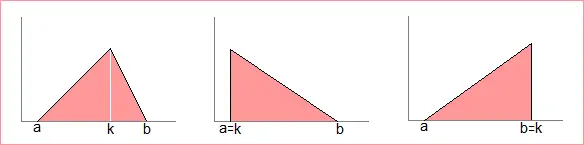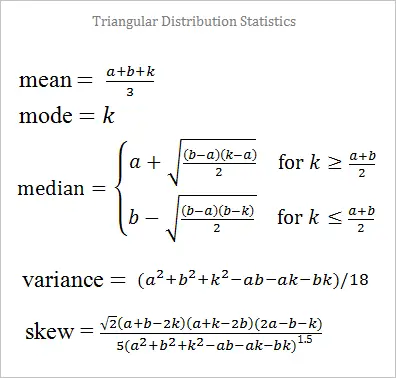# Triangle Distribution Random Variable Generator

The triangular distribution with parameters a, b, and k is bounded between a and b with a peak at k. It is often used in business and project management situations where not much information is known about a distribution except for the min, max, and mode. You can use the random number generator below to produce random variables from a triangular distribution. The parameter k may be equal to either a or b.

 a =     k =     b =     N = Decimal Precision integer tenths hundredths thousandths Display comma separated verticalThe mean, median, mode, variance, and skewness of the triangular distribution are elementary functions of a, b, and k:The triangular distribution arises when studying several uniformly distributed random variables. For example, if X and Y are uniform random variables from the interval [0, 1], then the arithmetic mean of X and Y has a triangular distribution with a = 0, k = 0.5, and b = 1. The absolute value of the difference between X and Y also has a triangular distribution; in this case a = k = 0 and b = 1.